# BMAT: Section 2 Physics [The Definitive Guide]

## Number of questions In BMAT Section 2

The ‘Scientific Knowledge and Applications’ is compromised of Biology, Chemistry, Physics and Mathematics questions.

In total there are 27 questions and weighting for each topic is shown in the table below.

TopicNumber Of Questions
Biology7
Chemistry7
Physics7
Mathematics6
Total27

You have 30 minutes to complete all of Section 2 which gives you 66 seconds per question.

## BMAT Section 2 Time Pressure

Time pressure is a skill that will be tested throughout the BMAT, including the Physics questions.

You will therefore need to become familiar with these types of conditions. Here are a few things to watch out for:

• The Physics questions in particular require you to quickly recall various equations and reactions.
• You should also get used to analysing data quickly and deciphering exactly what the questions are looking for.
• You should become confident doing calculations without using a calculator.
• Becoming familiar with the test under exam conditions is extremely valuable – you’ll get a feel for the timings of the real test.

## BMAT Section 2: Physics Specification

All examinable content for the Physics questions in BMAT Section 2 are shown below. This has been adapted from Cambridge Assessment Admission Testing’s BMAT content specification.

 P1.1 Electrostatics: a. Know and understand that insulators can be charged by friction b. Know and understand that charging is caused by gain or loss of electrons c. Know and understand that like charges repel and unlike charges attract d. Understand applications and hazards associated with electrostatics, including the role of earthing P1.2 Electric circuits: a. Know and recognise the basic circuit symbols and diagrams, including: cell, battery, light source, resistor, variable resistor, ammeter, voltmeter, switch, diode b. Understand the difference between alternating current (ac) and direct current (dc) c. Understand the difference between conductors and insulators, and recall examples of each type d. Know and be able to apply: current = charge/time, I = V / I e. Know and understand the use of voltmeters and ammeters f. Know and be able to apply: resistance = voltage/current, R = V / I g. Recall and interpret V–I graphs for a fixed resistor and a filament lamp h. Know the properties of NTC (negative temperature coefficient) thermistors, LDRs (light-dependent resistors) and ideal diodes i. Know and understand the current and voltage rules for series and parallel circuits j. Calculate the total resistance for resistor combinations in series k. Understand that the total resistance of a parallel combination is less than that of any individual resistor l. Know and be able to apply: voltage = energy/charge, V = E / Q m. Know and be able to apply: power = current × voltage, P = IV = I2 R n. Know and be able to apply: energy transfer = power × time, E =VIt
 P2.1 Properties of magnets: a. Know and be able to use the terms north pole, south pole, attraction and repulsion b. Know the magnetic field pattern around a bar magnet (including direction) c. Understand the difference between soft and hard magnetic materials (e.g. iron and steel) d. Qualitatively understand induced magnetism P2.2 Magnetic field due to an electric current: a. Know and understand the magnetic effect of a current b. Know the magnetic field patterns around current-carrying wires (including direction) for straight wires and coils/solenoids c. Know and understand the factors affecting magnetic field strength around a wire d. Understand the difference between permanent magnets and electromagnets P2.3 The motor effect: a. Know that a wire carrying a current in a magnetic field can experience a force b. Know the factors affecting the direction of a force on a wire in a magnetic field (including the left-hand rule) c. Know the factors affecting the magnitude of the force on a wire in a magnetic field d. Know and be able to apply F=BIL for a straight wire at right angles to a uniform magnetic field e. Know and understand the construction and operation of a dc motor, including factors affecting the magnitude of the force produced f. Understand applications of electromagnets P2.4 Electromagnetic induction: a. Know and understand that a voltage is induced when a wire cuts magnetic field lines, or when a magnetic field changes b. Know the factors affecting the magnitude of an induced voltage c. Know the factors affecting the direction of an induced voltage d. Understand the operation of an ac generator, including factors affecting the output voltage e. Interpret the graphical representation of the output voltage of a simple ac generator f. Understand applications of electromagnetic induction P2.5 Transformers: a. Know and understand the terms step-up transformer and step-down transformer b. Know and use the relationship between the number of turns on the primary and secondary coils, and the voltage ratio: Vp / Vs = np / ns c. Know that a consequence of 100% efficiency is total transfer of electrical power, and that this gives rise to the following relationship: VpIp = VsIs. Know and use this relationship to solve problems d. Understand power transmission, including calculating losses during transmission and the need for high voltage
 P3.1 Kinematics: a. Know and understand the difference between scalar and vector quantities b. Know and understand the difference between distance and displacement and between speed and velocity c. Know and be able to apply: speed = distance / time , velocity = change in displacement / time d. Know and be able to apply: acceleration = change in velocity / time e. Interpret distance–time, displacement–time, speed–time and velocity–time graphs f. Perform calculations using gradients and areas under graphs g. Know and be able to apply: average speed = total distance / time h. Know and be able to apply the equation of motion: v2 – u2 = 2as P3.2 Forces: a. Understand that there are different types of force, including weight, normal contact, drag (including air resistance), friction, magnetic, electrostatic, thrust, upthrust, lift and tension b. Know and understand the factors that can affect the magnitude and direction of the forces in 3.2a c. Draw and interpret force diagrams d. Qualitatively understand resultant force, with calculations in one dimension P3.3 Force and extension: a. Interpret force–extension graphs b. Understand elastic and inelastic extension, and elastic limits c. Know and be able to apply Hooke’s law (F = kx), and understand the meaning of the limit of proportionality d. Understand energy stored in a stretched spring as: E = 1/2 Fx = 1/2 kx2 P3.4 Newton’s laws: a. Know and understand Newton’s first law as: ‘a body will remain at rest or in a state of uniform motion in a straight line unless acted on by a resultant external force’ b. Understand mass as a property that resists change in motion (inertia) c. Know and understand Newton’s second law as: force = mass × acceleration d. Know and understand Newton’s third law as: ‘if body A exerts a force on body B then body B exerts an equal and opposite force of the same type on body A’ P3.5 Mass and weight: a. Know and understand the difference between mass and weight b. Know and be able to apply gravitational field strength, g, approximated as 10 Nkg–1 on Earth c. Know and be able to apply the relationship between mass and weight: w = mg d. Understand free-fall acceleration e. Know the factors affecting air resistance f. Understand terminal velocity and the forces involved P3.6 Momentum: a. Know and be able to apply: momentum = mass × velocity, p = mv b. Know and be able to use the law of conservation of momentum in calculations in one dimension c. Know and be able to apply: force = rate of change of momentum P3.7 Energy: a. Know and be able to apply: work = force × distance moved (in direction of force) b. Understand work done as a transfer of energy c. Know and be able to apply: gravitational potential energy = mgh, where h is the difference in height of the object d. Know and be able to apply: kinetic energy = 1/2mv2 e. Know and be able to apply: power = energy transfer / time f. Know and be able to use in calculations the law of conservation of energy g. Understand the concepts of useful energy and wasted energy h. Know and be able to apply: percentage efficiency = useful output / total output x 100
 P4.1 Conduction: a. Know and understand thermal conductors and insulators, with examples b. Know and be able to apply factors affecting rate of conduction P4.2 Convection: a. Understand and be able to apply the effect of temperature on density of fluid b. Understand and be able to apply fluid flow caused by differences in density P4.3 Thermal radiation: a. Understand thermal radiation as electromagnetic waves in the infrared region b. Know and be able to apply absorption and emission of radiation c. Know and be able to apply factors affecting rate of absorption and emission of thermal radiation P4.4 Heat capacity: a. Understand the effect of energy transferred to or from an object on its temperature b. Know and be able to apply: specific heat capacity = thermal energy / mass x temprature change where temperature is measured in °C and specific heat capacity, c, is measured in J kg–1 °C–1
 P5.1 States of matter: a. Know the characteristic properties of solids, liquids and gases b. Know and be able to apply particle models of solids, liquids and gases c. Know and be able to explain properties of solids, liquids and gases in terms of particle motion and the forces and distances between the particles P5.2 Ideal gases: a. Be able to explain pressure and temperature in terms of the behaviour of particles b. Understand and be able to apply the effect of pressure (P) on gas volume (V) constant temperature, i.e. PV = constant P5.3 State changes: a. Understand the terms melting point and boiling point b. Know and understand the terms latent heat of fusion and latent heat of vaporisation c. Know and be able to apply specific latent heat calculations. P5.4 Density: a. Know and be able to apply: density = mass / volume , p = m / v b. Understand the experimental determination of densities c. Be able to compare the densities of solids, liquids and gases P5.5 Pressure: a. Know and be able to apply: pressure = force / area b. Know and be able to apply: hydrostatic pressure = hρg, where h is the height, or depth, of the liquid
 P6.1 Wave properties: a. Understand the transfer of energy without net movement of matter b. Know and understand transverse and longitudinal waves c. Know and understand the terms: peak, trough, compression and rarefaction. d. Recall examples of waves, including electromagnetic waves and sound e. Know and be able to use the terms: amplitude, wavelength, frequency and f. period g. Know and be able to apply: frequency = 1 / period , f = 1 / T h. Know and be able to apply: wave speed = frequency × wavelength, v = fλ P6.2 Wave behaviour: a. Know and understand reflection at a surface b. Know and understand refraction at a boundary c. Know and understand the effect of reflection and refraction on the speed, frequency, wavelength and direction of waves d. Know and understand the analogy of reflection and refraction of light with that of water waves e. Know and understand the Doppler effect P6.3 Optics: a. Draw and interpret ray diagrams to describe reflection in plane mirrors b. Know and be able to apply: angle of incidence = angle of reflection c. Draw and interpret ray diagrams for refraction at a planar boundary d. Know and be able to interpret angle of incidence and angle of refraction e. Know and understand the effect of refraction on wave direction (away from or towards the normal) and speed (increasing or decreasing) P6.4 Sound waves: a. Understand the production of sound waves by a vibrating source b. Understand the need for a medium c. Understand qualitatively the relation of loudness to amplitude and pitch to frequency d. Know and understand longitudinal waves. e. Understand that reflection causes echoes e. Recall that the range of human hearing is 20Hz to 20 kHz f. Know and understand ultrasound and its uses (sonar and medical scanning) P6.5 Electromagnetic spectrum: a. Know and understand the nature and properties of electromagnetic waves (they are transverse waves and travel at the speed of light in a vacuum) b. Recall the component parts of the spectrum (radio waves, microwaves, IR, visible light, UV, X-rays, gamma) c. Understand the distinction of the component parts by different wavelengths and/or frequencies d. Recall the order of the component parts by wavelength and/or frequency e. Understand applications and hazards of the component parts of the electromagnetic spectrum
 P7.1 Atomic structure: a. Understand the atom in terms of protons, neutrons and electrons b. Know and be able to apply the nuclear model of atomic structure c. Know the relative charges and masses of protons, neutrons and electrons d. Understand and be able to use the terms atomic number and mass number e. Know and understand the term isotope f. Know and understand the term nuclide, and use nuclide notation g. Understand that ionisation is caused by the gain/loss of electrons P7.2 Radioactive decay: a. Know and understand that emissions arise from an unstable nucleus b. Know and understand the random nature of emissions c. Know and understand the differences between alpha, beta and gamma emission d. Know and understand the nature of alpha and beta particles, and gamma radiation e. Be able to use and interpret nuclear equations f. Know the effect of decay on atomic number and mass number P7.3 Ionising radiation: a. Know the relative penetrating abilities of alpha, beta and gamma radiation b. Know the relative ionising abilities of alpha, beta and gamma radiation c. Understand qualitatively the deflection of alpha, beta and gamma radiation in electric or magnetic fields d. Know and appreciate the existence of background radiation e. Understand the applications and hazards of ionising radiation P7.4 Half-life: a. Be able to interpret graphical representations of radioactive decay (including consideration of decay products) b. Understand the meaning of the term half-life c. Understand and be able to apply half-life calculations

You are also expected to be familiar with the following SI prefixes:

Prefix NameValue
Nano-10-9
Micro-10-6
Milli-10-3
Centi-10-2
Deci-10-1
Kilo-103
Mega-106
Giga-109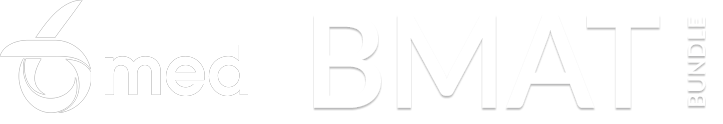## Revising For BMAT Physics Topics

Cambridge Assessment Admissions Testing’s website contains a useful preparation guide to ensure you are prepared for the Physics questions in ‘Scientific Knowledge and Applications’.

The Physics questions can also be prepared for by going through the above specification, adapted from Cambridge Assessment Admission Testing’s BMAT Content Specification.

If you find that you haven’t covered a specific area of the specification in your school lessons then you should find a suitable resource, like a Physics textbook or 6med’s BMAT resources.

Finally, 6med have a ton of BMAT resources available for candidates to use for their preparation. Our BMAT Bundle gives you access to everything we have on offer for the BMAT including a Crash Course and access to BMAT.Ninja (our online learning Dojo).

We’re probably biased, but we worked really hard on creating these resources and students feel they work, so if you’re interested – check them out. If money is holding you back, we offer generous bursaries, so please don’t let finances get in the way of your preparation.## BMAT Section 2 Question & Answer Types

All questions will be in a multiple-choice format, as mentioned previously. On the day you will have a question book and an answer sheet only. The answer sheet will look similar to this: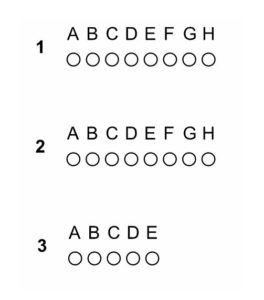As you can see, the number of options for an answer varies for each question.

You should shade the appropriate circle with a soft pencil so that you can change your answer if necessary.

Unfortunately, there are no marks for showing your workings out. The questions in Section 2 could test you on any area of the Physics specification listed above.

The type of questions could also vary greatly and include data analysis, calculations and true/false questioning.

## Fully Worked BMAT Physics Questions & Answers

In this part of the article we’ll work through three BMAT Physics practice questions with in-depth solutions.

If you want to work through the questions yourself first scroll with care!

### Question 1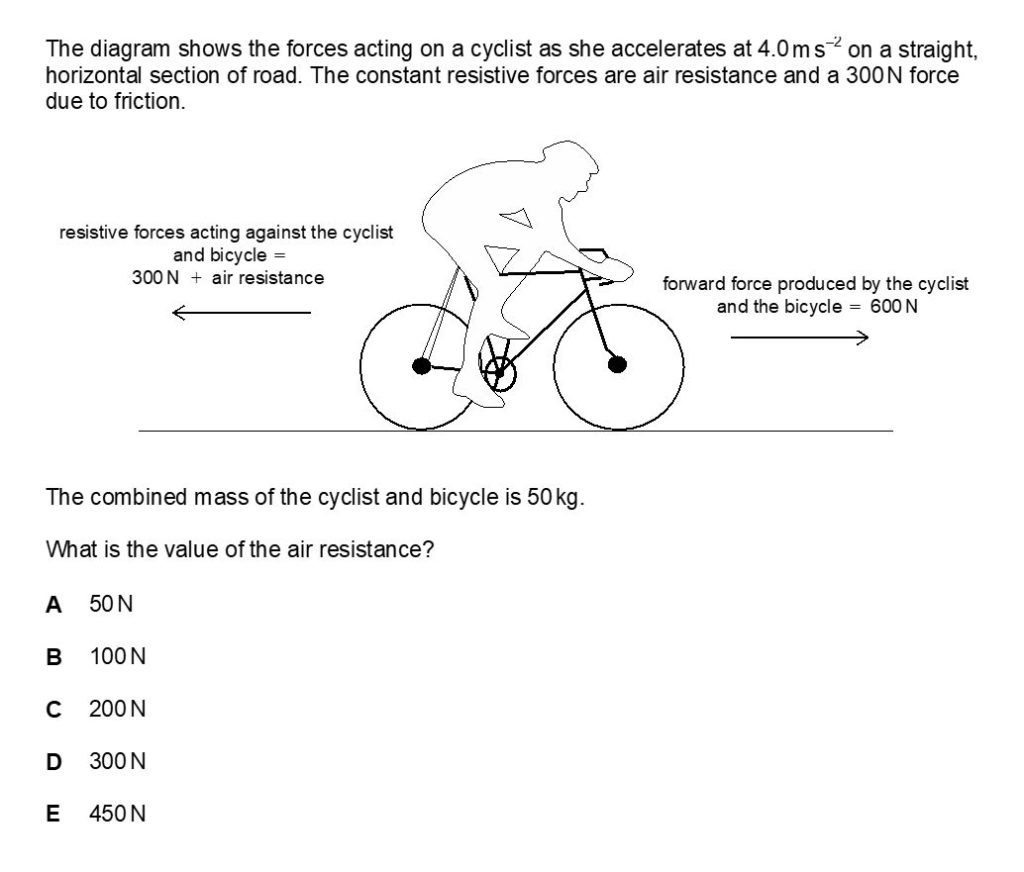### Question 1 Fully Worked Solution

The answer for this is B.

The equation needed here is A = F/M (A = acceleration (ms-2), F = force (N), M = mass (kg)).

The acceleration (4.0ms-2), mass (50kg) and some of the details for the force is provided.

We know that the forward force produced by the cyclist and the bicycle is 600N. We also know that the resistive forces acting against the cyclist and the bicycle without air resistance is 300N.

We need to calculate the air resistance. To fill in the equation:

A = F/M

4 = (600 – 300 – x)/50

300 – x = 200

x = 100

Therefore the air resistance is 100N totalling the resistive forces acting against the cyclist and bicycle to 400N.

### Question 2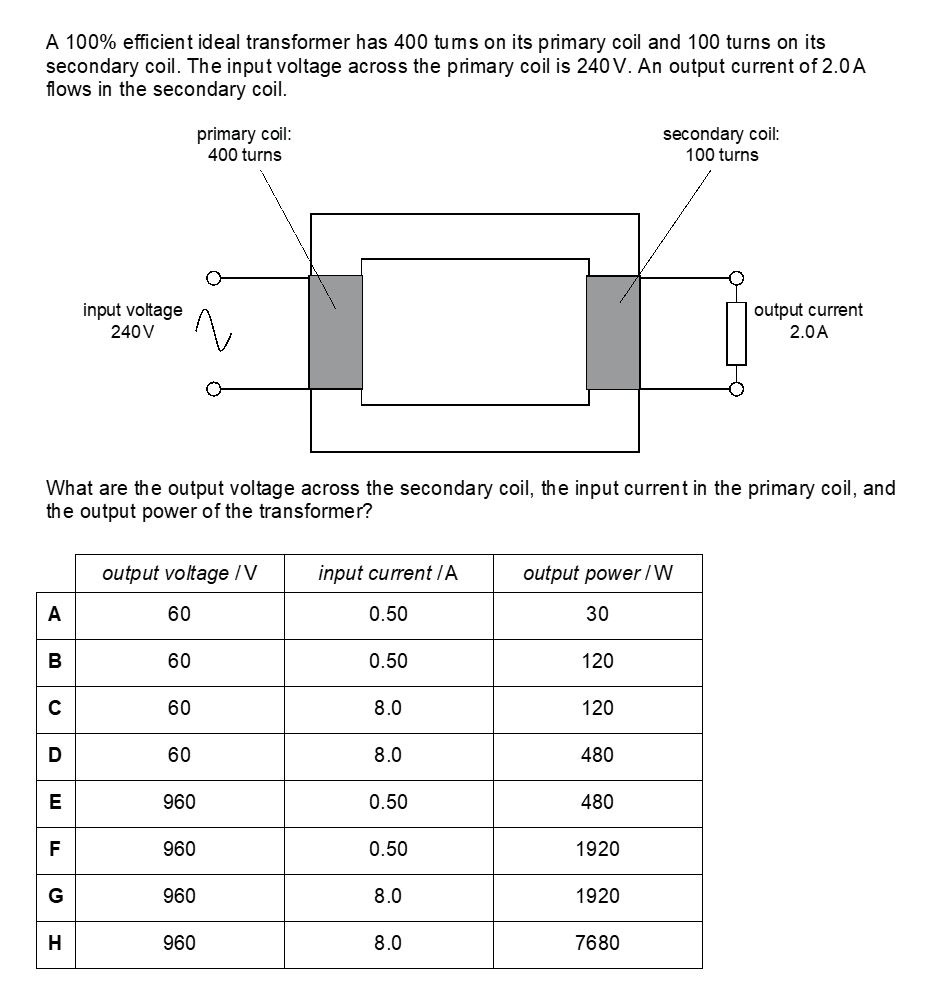### Question 2 Fully Worked Solution

The answer for this is B.

We first need to find out the output voltage. The equation needed here is Vp / Vs = np / ns.

Vp is the potential difference in the primary (input) coil in volts (V).

Vs is the potential difference in the secondary (output) coil in volts (V). np is the number of turns on the primary coil and ns is the number of turns on the secondary coil.

Vs = 240 x (100/400)

Vs = 60V

This means that the only possible answers left are A-D. We can easily find out the output power using the equation power = current x voltage. Power (W) = 2 x 60. Output power is 120W, which means that either B or C is the correct answer. To work out the input current the equation needed is:

60 x 2 = 240 x Ip

Ip = 120/240

Ip = 0.5A.

The correct answer is therefore B.

### Question 3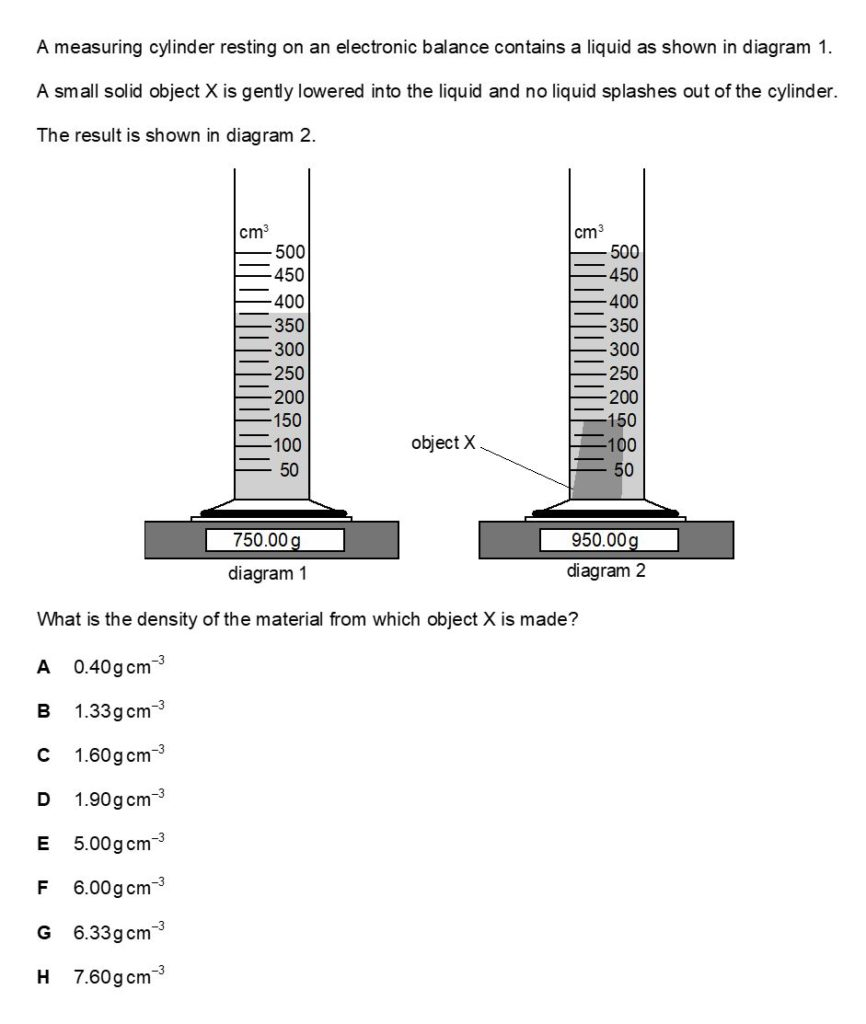### Question 3 Fully Worked Solution

The answer for this is C.

The equation needed here is D = M/V (D = density (g cm3), M = mass (g), V = volume (cm3)).

The mass can be calculated by seeing the difference on the scales (950 – 750 = 200g) before and after object X is lowered into the liquid).

The volume is can be calculated by seeing the difference in reading on the cylinder (500 – 375 = 125cm3).

Therefore the density of object X is 200/125 = 1.60g cm3.## BMAT Section 2 Scoring for Physics questions

Physics questions make up 7 of the total 27 questions in Section 2 of the BMAT.

Every question is worth one mark and there is no negative marking.

There is also no pass/fail threshold in the BMAT, therefore, it is advisable to try and attempt every question. Section 1 and 2 marks are combined to form a final mark, which is then converted to BMAT’s scale.

This scale runs from 1 (low) to 9 (high). The average test-taker scores 5.0 and exceptional candidates score 7.0 or above.

## Closing Tips for the BMAT Biology section

1

It’s worth going through the specification systematically and ticking what you know, and highlighting what you don’t know or aren’t comfortable with.

Focus on these topics to ensure you’ve got an even spread of knowledge for the exam as Cambridge Admissions Assessment can test anything on the specification.

2

Practice is key!

Practice is incredibly important if you want to do well in the Physics questions of the BMAT. More practice means more familiarity with the question types and exam as a whole.

3

Use worked solutions for tough questions.

Questions with worked solutions give you a valuable insight as to WHY a question is right or wrong – use them wherever you can.

Our BMAT Ninja platform has 1,400 practice questions with fully worked solutions for each of them so, if this method helps you, you can get access through the BMAT Bundle or individually.

And there we have it – the BMAT Physics portion from A-Z.

By no means is this the end of your preparation, but this article should give you a great starting point with which to continue your preparation.

If you feel like you need extra support, 6med are always on hand to help.